Manifold-Manifold Distance with Application to
Face Recognition based on Image Set

1Key Lab of Intelligent Information Processing, Chinese Academy of Sciences

2School of EE&CS, Peking University, Beijing, China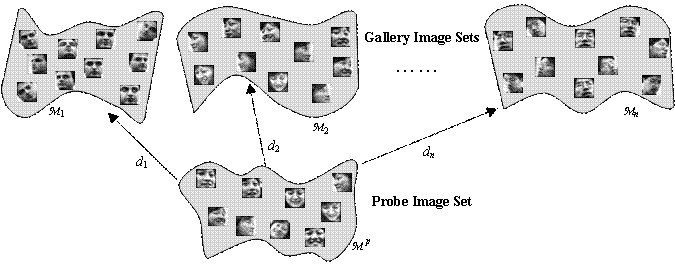Face Recognition based on Image Set (FRIS)

Abstract - In this paper, we address the problem of classifying image sets, each of which contains images belonging to the same class but covering large variations in, for instance, viewpoint and illumination. We innovatively formulate the problem as the computation of Manifold-Manifold Distance (MMD), i.e., calculating the distance between nonlinear manifolds each representing one image set. To compute MMD, we also propose a novel manifold learning approach, which expresses a manifold by a collection of local linear models, each depicted by a subspace. MMD is then converted to integrating the distances between pair of subspaces respectively from one of the involved manifolds.

The proposed MMD method is evaluated on the task of Face Recognition based on Image Set (FRIS). In FRIS, each known subject is enrolled with a set of facial images and modeled as a gallery manifold, while a testing subject is modeled as a probe manifold, which is then matched against all the gallery manifolds by MMD. Identification is achieved by seeking the minimum MMD. Experimental results on two public face databases, Honda/UCSD and CMU MoBo, demonstrate that the proposed MMD method outperforms the competing methods.

Publication:

Ruiping Wang, Shiguang Shan, Xilin Chen and Wen Gao

IEEE Conference on Computer Vision and Pattern Recognition (CVPR), Anchorage, Alaska, June 24-26, 2008. [paper]

Motivation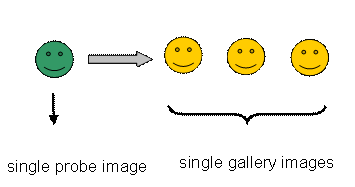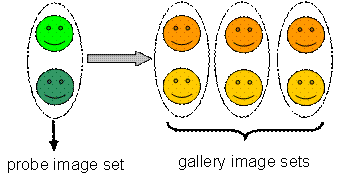Traditional recognition task Object Recognition based on Image Set (ORIS)

Problem Formulation

Ø        For visual object recognition, patterns can be represented in three possible levels:

n        Point (P): individual sample

n        Subspace (S): linear model spanned by some samples

n        Manifold (M): nonlinear low-dimensional embedding learned from a large number of samples

Ø        In some sense, the core of pattern classification is the distance computation among the three representations.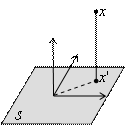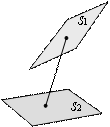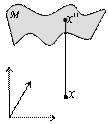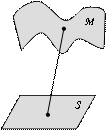point to point distance (PPD) point to subspace distance (PSD) subspace to subspace distance (SSD) point to manifold distance (PMD) subspace to manifold distance (SMD) manifold to manifold distance (MMD)

Manifold to Manifold Distance (MMD)

Ø        Basic ideas

n        Manifold can be modeled by a collection of local linear subspaces.

n        The distances associated with manifold can be converted to those defined on subspaces.

n        Formally, we denote the component subspaces of a manifold M  by Ci  , and express a manifold as:Ø        Our approach

n        MMD is converted to the integration of distances between pair of subspaces.

n        For the ORIS task, the most effective solution is to find the common views and measure the similarity of those parts of data.

n        Therefore, we define MMD by the closest subspace pair from the two manifolds.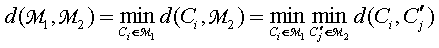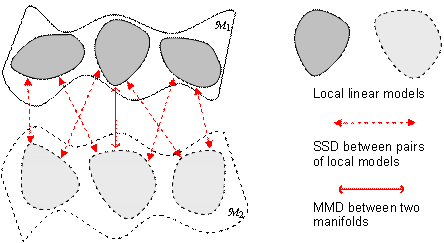Conceptual illustration

Ø        Discussion

n        We measure the similarity between two manifolds by the similarity between their best suited local models.

n        While many others may be explored, however, for the ORIS task, our definition is believed to be one of the most appropriate.

Local linear model construction

Ø        Maximal Linear Patch (MLP)

n        Linear criterion: geodesic distance is as close to Euclidean distance as possible in the patch, which guarantees the near linearity of the patch.

n        Maximal criterion: the patch size is maximized such that any appending of additional data point would violate the linear criterion.

n        Each MLP is then characterized by a linear subspace using PCA.

Ø        One-shot algorithm to construct MLPs

n        Basic idea: each new MLP is stemmed from a seed point gradually until the linear constraint is broken.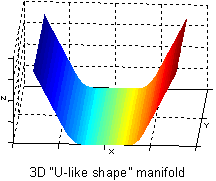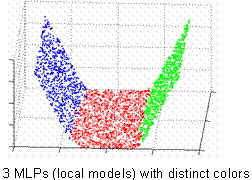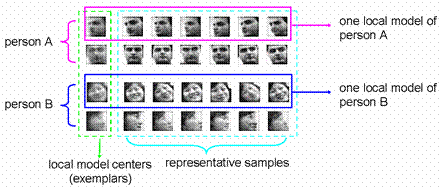Illustration of MLP on a toy example Local models constructed from real face dataset

Local linear model distance

For two subspaces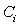and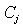Ø        Denote their exemplars by: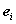,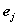, and bases by: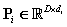,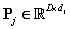.

Ø        Define variation distance measure by the average of canonical correlations: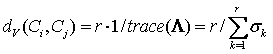Ø        Define exemplar distance measure by the correlation of the two exemplars: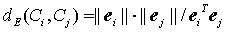Ø        Our definition of SSD (local linear model distance):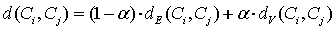,     (is a weight parameter)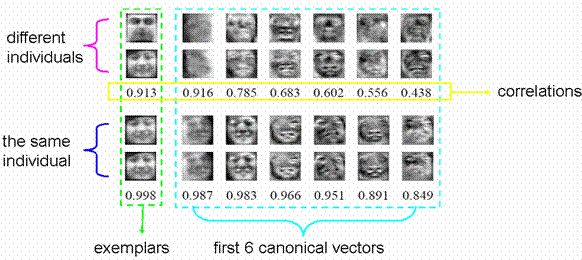Local model similarity by using our definition of SSD

Experimental Results

Ø        Experimental setting on FRIS

n        Each known subject (enrolled with a set of images) is modeled as a gallery manifold.

n        A testing subject is modeled as a probe manifold, which is then matched against all the gallery manifolds by MMD.

n        Identification is achieved by seeking the minimum MMD.

Ø        Datasets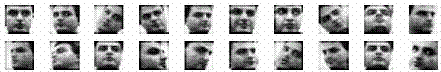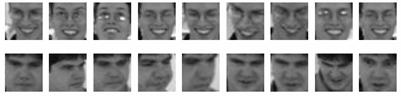Examples from Honda/UCSD Examples from CMU MoBo

 Table 1. Two public datasets for FRIS task Database Persons Videos Image Size Honda/UCSD 20 59 20*20 CMU MoBo 25 100 30*30

Ø        Comparative methods

n        Nearest neighbor (NN) matching in (i) Eigenface, and (ii) Fisherface,

n        NN matching in LLE + k-means clustering, which is a typical exemplar-based method,

n        Mutual Subspace Method (MSM), which is a typical variation-based method,

n        The proposed  MMD method.

Ø        Identification results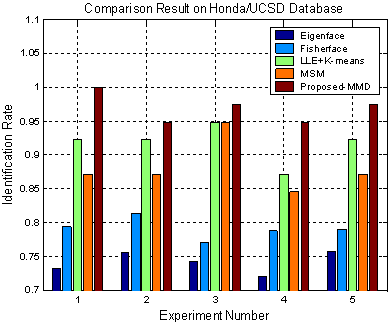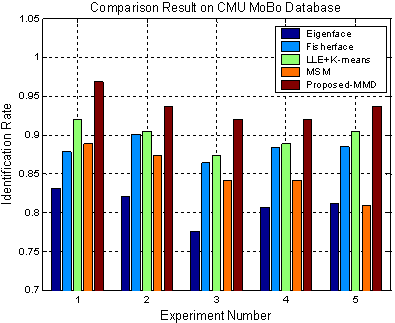Conclusion

Ø        We formally and explicitly formulate the ORIS task as the computation of what we name manifold to manifold distance (MMD).

Ø        We propose a formal definition of MMD, and present several technical contributions for its computation, i.e.,

n        The local linear model construction method

n        A more effective subspace distance measure definition.

Ø        The proposed MMD method is applied to Face Recognition with Image Set (FRIS) problem, and impressive results are achieved.